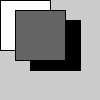Name

### pushMatrix()

Examples```fill(255)
rect(0, 0, 50, 50)# White rectangle
pushMatrix()
translate(30, 20)
fill(0)
rect(0, 0, 50, 50)# Black rectangle
popMatrix()
fill(100)
rect(15, 10, 50, 50)# Gray rectangle
```
Description Pushes the current transformation matrix onto the matrix stack. Understanding pushMatrix() and popMatrix() requires understanding the concept of a matrix stack. The pushMatrix() function saves the current coordinate system to the stack and popMatrix() restores the prior coordinate system. pushMatrix() and popMatrix() are used in conjuction with the other transformation functions and may be embedded to control the scope of the transformations.
Syntax
```pushMatrix()
```
Related popMatrix()
translate()
rotate()
rotateX()
rotateY()
rotateZ()

Updated on Mon Sep 21 15:53:25 2020.

If you see any errors or have comments, please let us know.